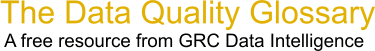Choose Index below for a list of all words and phrases defined in this glossary.

# Correlation Coefficient (r)

index | Index

## Correlation Coefficient (r) - definitions

Correlation Coefficient (r) - The correlation coefficient quantifies the degree of linear association between two variables. It is typically denoted by r and will have a value ranging between negative 1 and positive 1.

[Category=Data Quality ]

Source: iSixSigma, 11 January 2011 09:11:00, http://www.isixsigma.com/index.php?option=com_glossaryThese advertisers support this free service

correlation coefficient - A correlation coefficient is a statistical measure of the degree to which changes to the value of one variable predict change to the value of another. In positively correlated variables, the value increases or decreases in tandem. In negatively correlated variables, the value of one increases as the value of the other decreases.

Correlation coefficients are expressed as values between +1 and -1. A coefficient of +1 indicates a perfect positive correlation: A change in the value of one variable will predict a change in the same direction in the second variable. A coefficient of -1 indicates a perfect negative correlation: A change in the value of one variable predicts a change in the opposite direction in the second variable. Lesser degrees of correlation are expressed as non-zero decimals. A coefficient of zero indicates there is no discernable relationship between fluctuations of the variables.

[Category=Data Management ]

Source: WhatIs.com, 15 July 2013 09:02:01, http://whatis.techtarget.com/glossary/Data-and-Data-ManagementData Quality Glossary.  A free resource from GRC Data Intelligence. For comments, questions or feedback: dqglossary@grcdi.nl